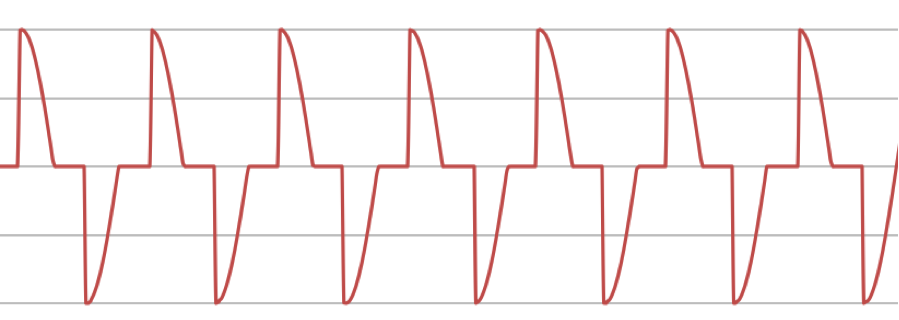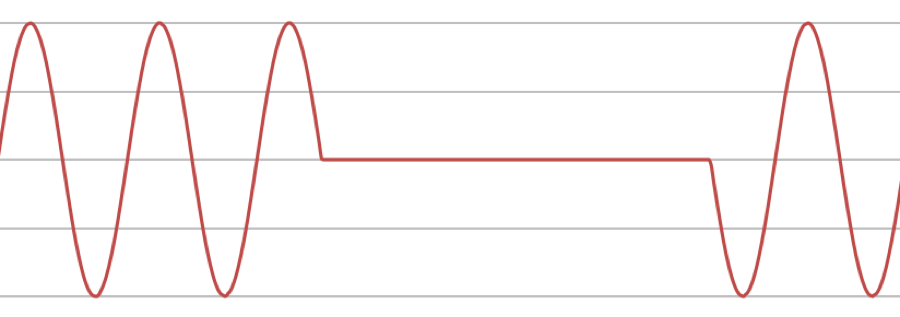# Control / Firing Modes

The power delivered to a load may be regulated or proportioned by SCR power controllers using either the phase-angle or the integral cycle (zero-cross voltage switching) control mode. Each control mode has its own specific advantages and disadvantages and each application should be reviewed to determine the most compatible mode of control.

## Phase-angle

In phase-angle control, each SCR of the back-to-back pair is turned on for a variable portion of the half-cycle that it conducts. Power is regulated by advancing or delaying the point at which the SCR is turned ON within each half cycle. Light dimmers are an example of phase-angle control.

Phase-angle control provides a very fine resolution of power and is used to control fast responding loads such as tungsten-filament lamps or loads in which the resistance changes as a function of temperature. Phase-angle control is required if the load is transformer-coupled or inductive.

Phase-angle controllers are typically more expensive than zero-cross controllers because the phase-angle circuit requires more sophistication than does a zero-cross circuit. Phase-angle control of three-phase power requires SCR's in all three legs and is appreciably more expensive than zero-cross control which only requires SCR's in two of the three legs.## Zero-cross

The term zero-cross or synchronous operation of SCR's is derived from the fact that the SCR's are turned on only when the instantaneous value of the sinusoidal waveform is zero. In zero-cross operation power is applied for a number of continuous half-cycles and then removed for a number of half-cycles to achieve the desired load power in the same manner as power would be controlled with a mechanical switching device. The difference is that the SCR controllers always switch power when the instantaneous value of the applied voltage is zero. Also, the frequency of the on-off cycles can be extremely fast because there is no limit to the number of switching operations the SCR can perform.

Zero-cross controllers can provide two rather distinctively different types of control. Switching large amounts of current can result in line voltage variations which affect ambient lighting or other equipment. Time proportioning control is sometimes used to reduce this effect. The disadvantage is that power is applied in longer bursts which can in turn cause control problems and shorten heater life.

Distributive control is typically somewhat less expensive, provides a much faster cycle rate giving better controllability and longer heater life. It can also be used with much faster responding loads than can time proportioning.## Zero-cross time proportioning

Zero-cross time proportion control is accomplished with a fixed or constant time base, therefore the total of power "ON" time and power "OFF" time is always equal to a fixed value.

For example, if the time base is ten seconds and the desired power is 50%, then power is applied for 5 seconds and removed for 5 seconds. If the desired power were 25% then power is applied for 2.5 seconds and removed for the remaining period of 7.5 seconds.

The disadvantage of time proportioning particularly as the time base is increased is that the load temperature varies considerably between the on-off cycles. This can shorten the life of heater elements and decrease the ability to obtain precise process control.

## Distributive Zero-cross

Distributive zero-cross does not use a fixed or constant time base as is used in time proportioning. The technique used by Control Concepts applies load power for 3 electrical cycles and removes load power for 3 electrical cycles at 50% power.

At lower power requirements the controller will apply power for 3 electrical cycles and is then off for the appropriate number of electrical cycles. For example, at 25% power the controller is on for 3 electrical cycles and off for 9 electrical cycles, or on for 3 electrical cycles out of 12.

At higher power levels the controller is off for three electrical cycles and on for the appropriate number of electrical cycles. For example at 75% power the controller is on for nine electrical cycles and off for 3 electrical cycles.

The Control Concepts distributive control technique combines power pulses of short duration to obtain the exact power level proportional to the command or set point signal. For example, 60% power is achieved by combining two power pulses. The first power pulse consists of 4 on cycles and 3 off cycles; the second power pulse consists of 5 on cycles and 3 off cycles, providing a total of 9 on cycles during 15 cycles.

Of course, such rapid, short bursts of power would be impossible with mechanical contactors. Zero-cross control is typically less expensive than phase-angle control and generates fewer harmonics. However, zero-cross can only be used to control power to resistive loads that do not change appreciably with temperature or time and which are directly-coupled (no transformer between the SCR controller and the load).

Zero-cross control of large power levels can cause supply voltage fluctuations resulting in ambient lighting fluctuations or other problems and can cause overheating of transformers supplying power to the controller and load.